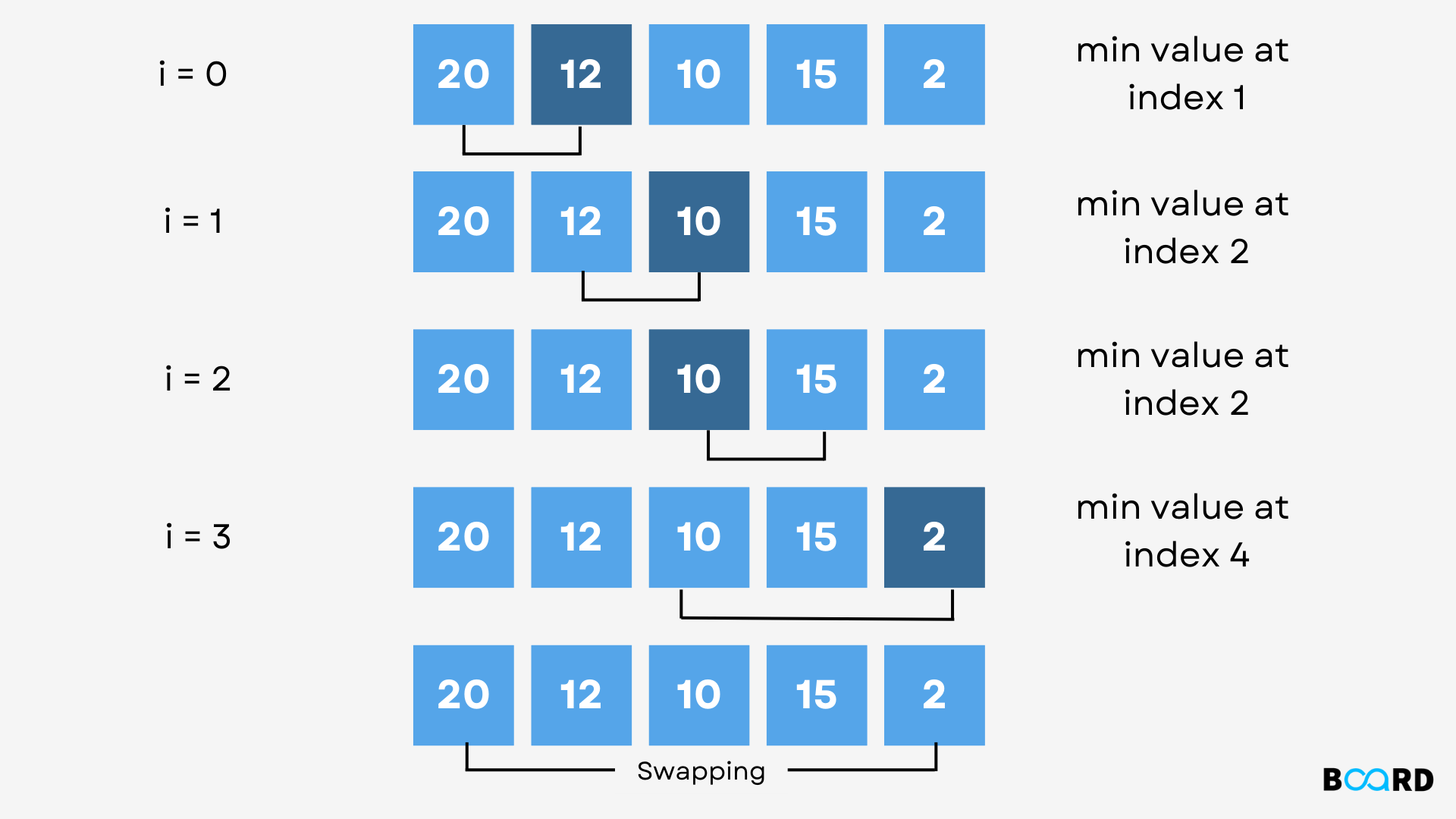# Selection Sort In Python## Overview

In this article, we shall learn about the selection sorting algorithm in Python. Selection sorting is an algorithm that sorts a given array by repeatedly searching for the smallest/largest element (depending upon the order of sorting) in an unsorted portion of the array, and then placing the found element at the start.

## Scope

• The concept of selection sorting.
• Implementing selection sort in Python.
• Time complexity of selection sort.
• Space complexity of selection sort.

## Concept

The selection sort algorithm operates by maintaining a partition of the given array into the following two arrays, at any point:

1. The subarray which is already sorted.
2. The remaining subarray, which has yet not been sorted.
In each of its iterations, the selection sort finds the smallest/largest element in the unsorted subarray and moves it to the sorted subarray.

Image Courtesy: technologystrive

## Implementation

 def selectionSorting(arr, n):    for index in range(n):        mn = index        for j in range(index + 1, n):            if arr[j] < arr[mn]:                mn = j        (arr[index], arr[mn]) = (arr[mn], arr[index]) arr = [-1, 0, 10, 7, 5, -6, -19, 23]sz = len(arr)selectionSorting(arr, sz)print('The selection sorted array is :')print(arr)

Output

 The selection sorted array is :[-19, -6, -1, 0, 5, 7, 10, 23]
write your code here: Coding Playground

O(n2)

O(1)